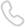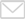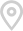﻿ High Speed Characteristics - T&S Communication Co, Ltd.Products

# High Speed Characteristics

 Parameter Symbol Min Typical Max Unit Note Differential Impedance TDR 90 100 110 Ώ - Insertion loss SDD21 -16.06 - - dB At 13.28 GHz Differential Return Loss SDD11SDD22 - - See 1 dB At 0.05 to 4.1 GHz - - See 2 dB At 4.1 to 19 GHz Common-mode to common-mode output return loss SCC11SCC22 - - -2 dB At 0.2 to 19 GHz Differential to common-modereturn loss SCD11SCD22 - - See 3 dB At 0.01 to 12.89 GHz - - See 4 At 12.89 to 19 GHz Differential to common ModeConversion Loss SCD21-IL - - -10 dB At 0.01 to 12.89 GHz - - See 5 At 12.89 to 15.7 GHz - - -6.3 At 15.7 to 19 GHz

Notes:

1. Reflection Coefficient given by equation SDD11(dB) < -16.5 + 2 × SQRT(f ), with f in GHz

2. Reflection Coefficient given by equation SDD11(dB) < -10.66 + 14 ×logo10/5.5, with f in GHz

3. Reflection Coefficient given by equation SCD11(dB) < -22 + (20/25.78)*f, with f in GHz

4. Reflection Coefficient given by equation SCD11(dB) < -15 + (6/25.78)*f, with f in GHz

Reflection Coefficient given by equation SCD21(dB) < -27 + (29/22)*f, with f in GHz

Recommended Products
Latest News & Blog
•Call us on:
0755-32983688
•Email Us:
info@china-tscom.com
•8 Jinxiu Middle Road,
Pingshan, Shenzhen, Guangdong,
518118, China